# 33. Excel – Gage R&R X Bar Setup

In this section, the excel setup from X Bar method for Gage R&R. For the equation setup and calculation limits, please refer to the Gage R&R Link for equation and constant setup.

Before initiating the Excel setup, the Excel file generated will be broken down into three sections. The Setup Section, Data Section and Graph Section for this particular gage R&R setup.

*Remark: All constant or calculated values are stored in single cell or pre-named under name manager, so the actual formula will be depending on which cell the required elements are stored or named.

Before collecting the gage R&R calculation and graph execution, The setup page will have critical input and essential parameters to perform gage R&R calculation for the index along with variation chart.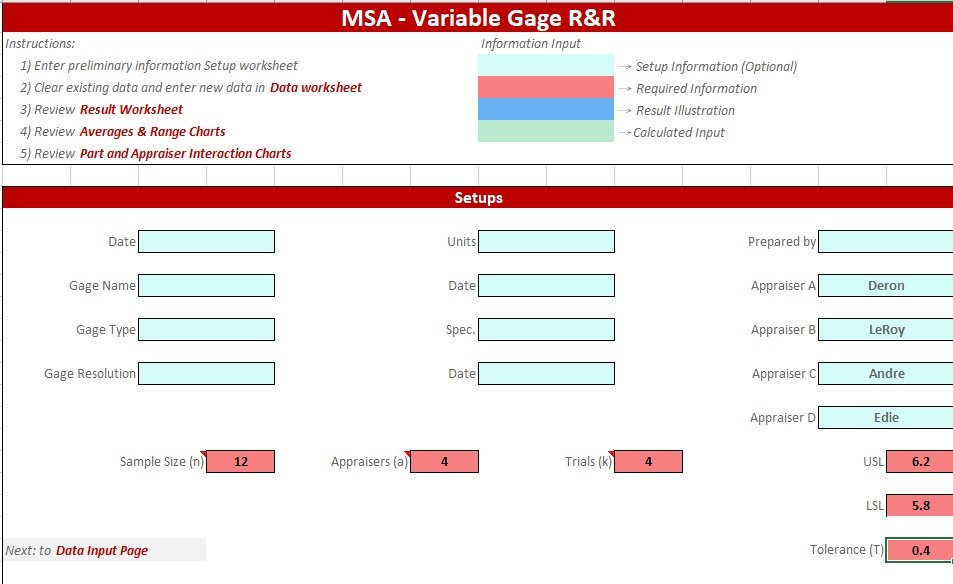MSA Setup Page

For the setup, 4 aspects shall be stored since it will impact the coefficient selection.

After the initial setup is completed for the numbers, then the data section should be implemented as the follwing format.Gage R&R Data Format

As seen in the data table, each appraiser will have maximum of 5 trials per sample and the following layout will have the following information.

The exact way to translate these calculation in Excel expression is listed below with caution remarks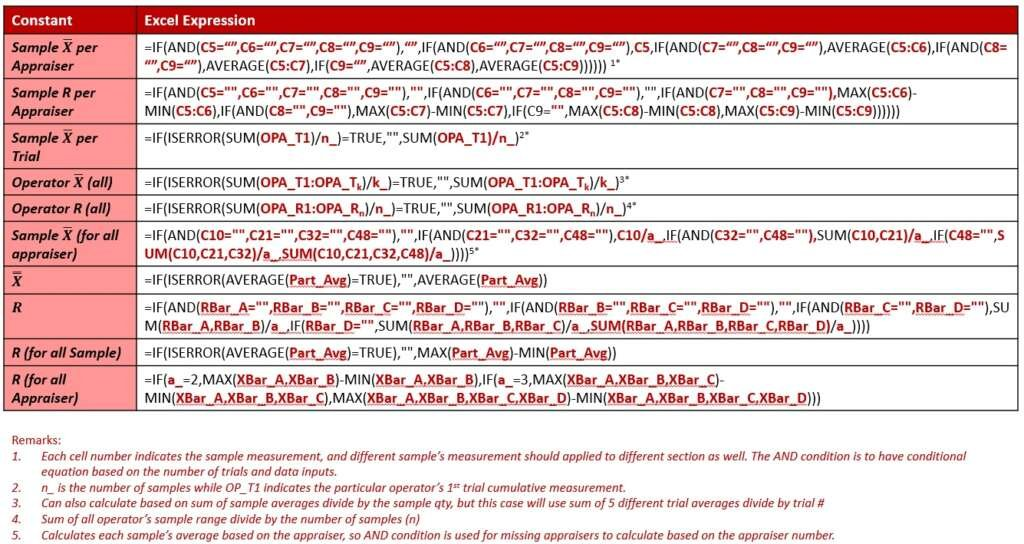Gage R&R Excel Expression

Once the data expression is completed, then the result section can be calculated and displayed below for setup.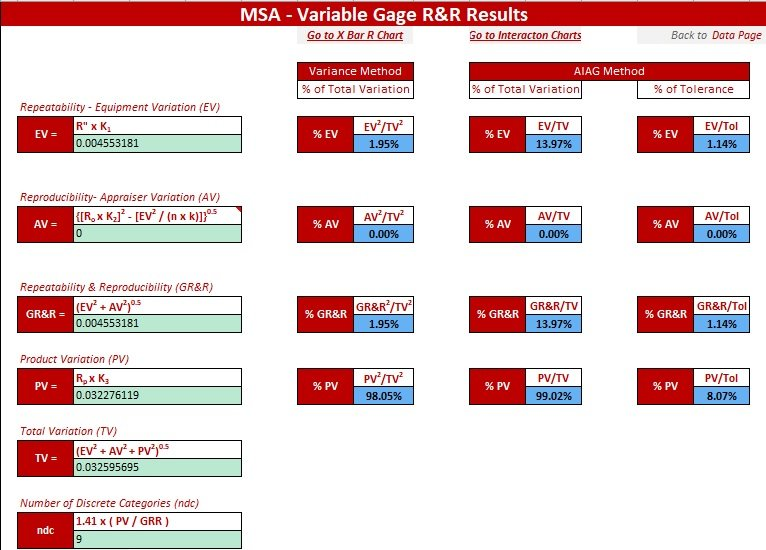Gage R&R Result Layout

The Result’s Excel expression is listed in the following table for calculation reference.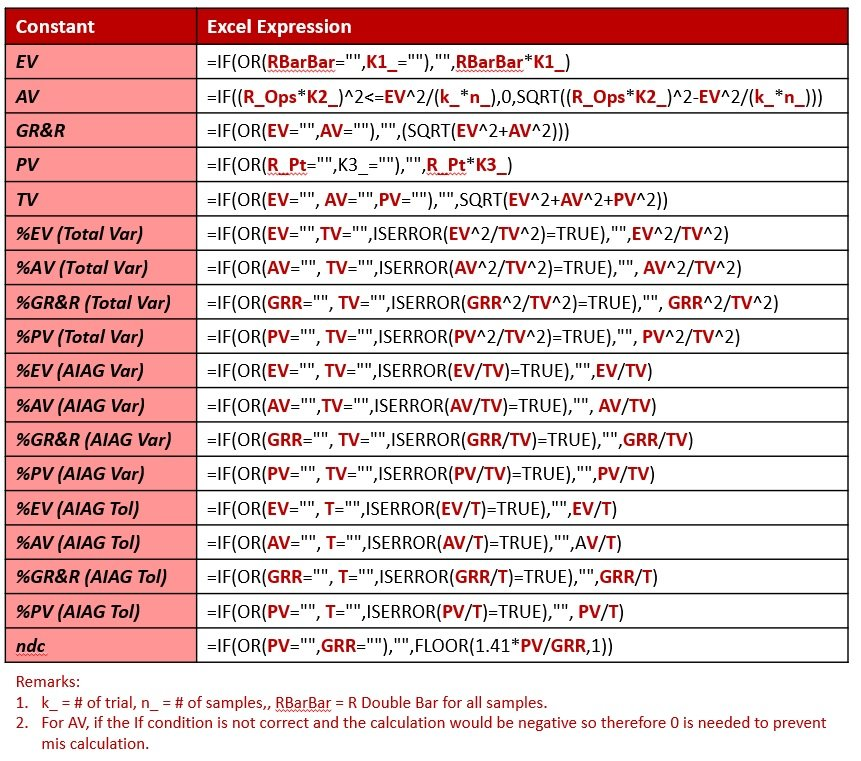Gage R&R Result Expression Setup

For the K1, K2 and K3 constant, a separate constant page is store and calculated below, and the representation of K1, K2 and K3 is referred below:

And the following table shows how to allocate the d2 constants and calculating appropriate setup for K1, K2 and K3.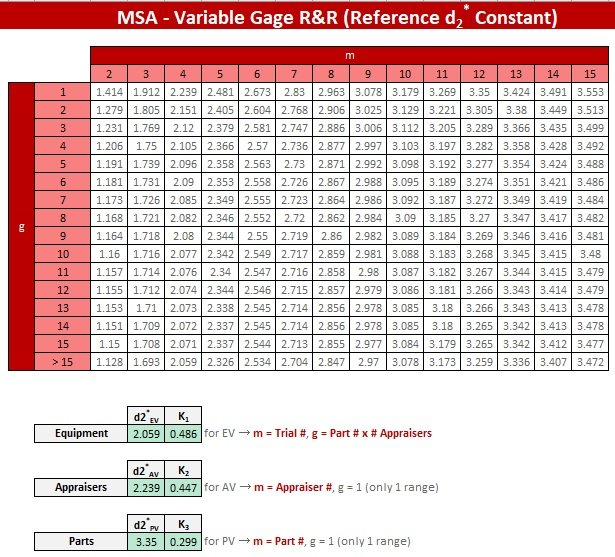d2 Constant Setup Cell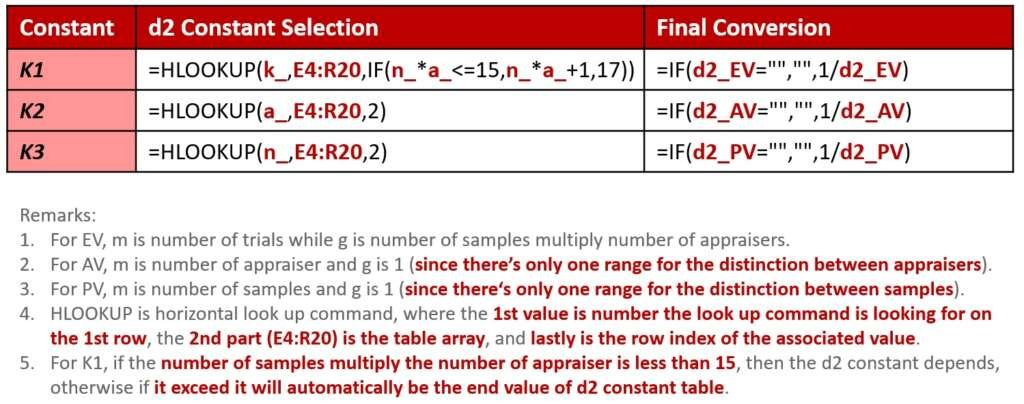K1, K2 and K3 Calculation’s Excel Expression

Regarding the result description and criteria fit in, please check the commands below for the PV% and GRR% acceptance standard expression.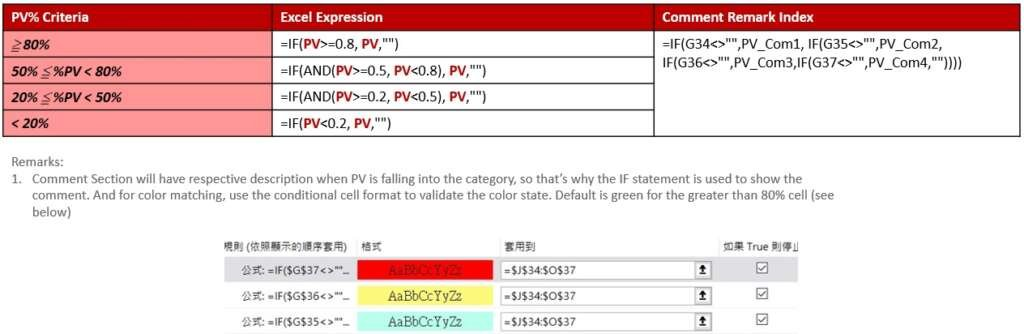PV% Excel Criteria Calculation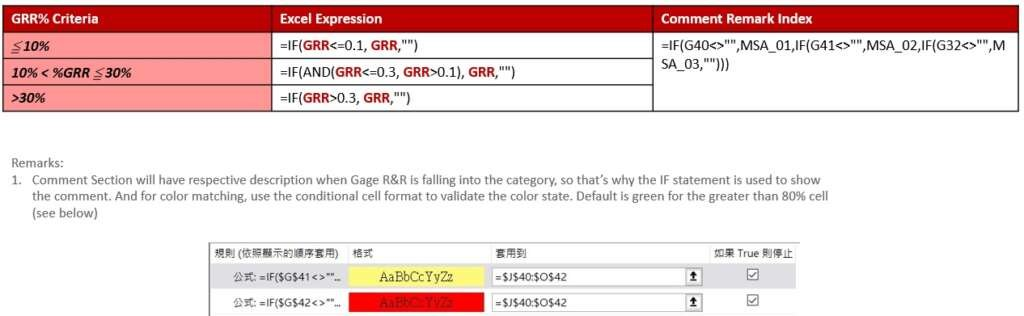GRR% Excel Criteria Calculation

After the results are displayed, then it’s the graphing section to visualize the plots. And for the graphs, the charts can be observed by the following aspects.

For the Variance Chart, please refer to the following Excel expression for plotting.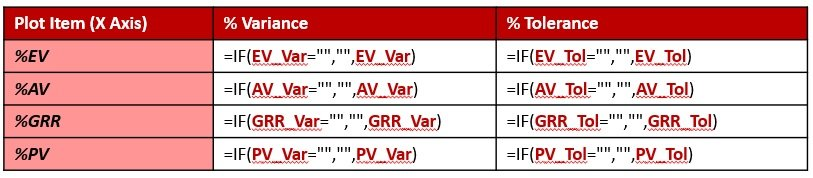Plot Cell and Layout for the Variance Plot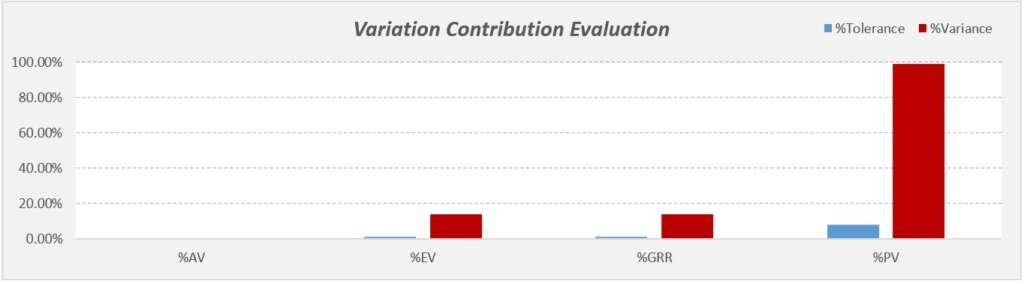Variation Contribution Plot Example

For the X Bar and R charts, please refer to the following table expression for plotting, where the left hand side is for the sample numbers respectively while the top axis is for appraiser’s data along with X” and responsive control limits (see quantitative control chart link for the calculation reference).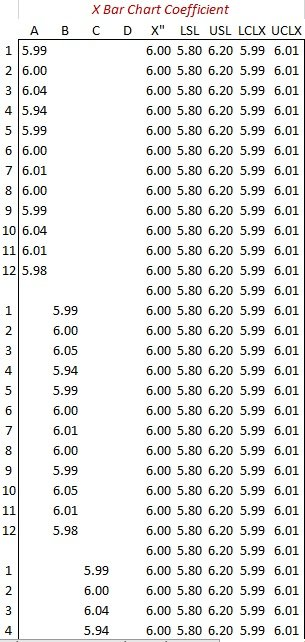X Bar Chart Table Expression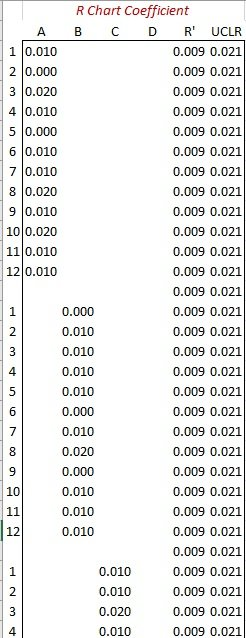R Chart Table Expression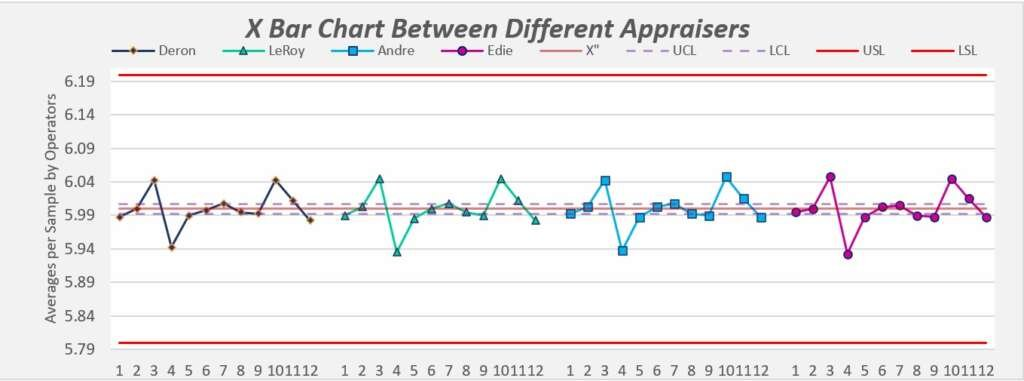X Bar Chart for the measurements in each appraiser’s average per sample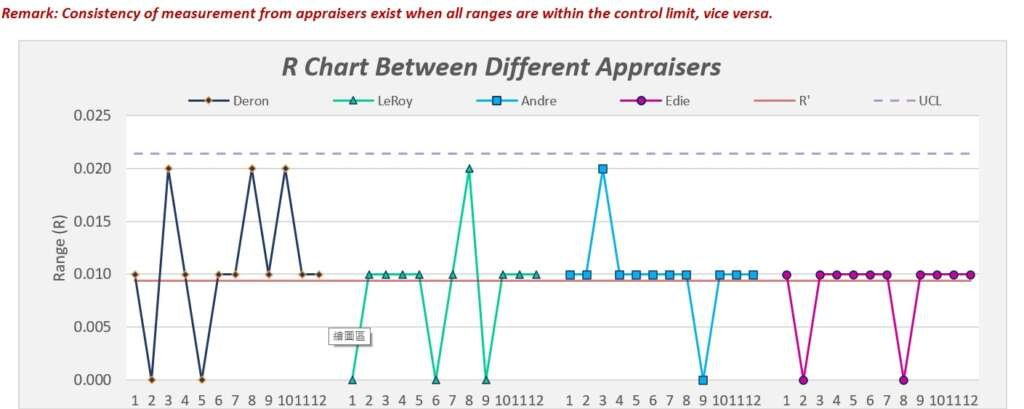R Chart for the measurements in each appraiser’s average per sample

Lastly, the interaction chart between operators are plotting the operators or sample as x axis while plot each sample’s average with all the individual trial data to see the trend for each sample or operators. This is to see if there’s any clear variance between appraiser’s measurement method along with sample comparison.

And since there’s a lot of data needs to be duplicated into graphing the forms, so I use the OFFSET expression and  return the value based on the horizontal (track column counts) for data setup.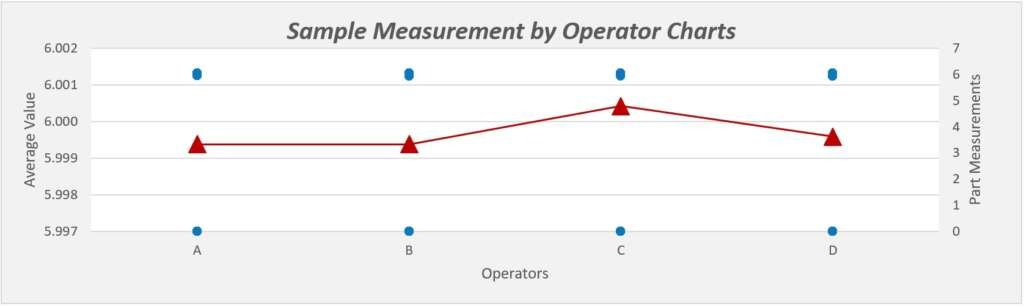Sample Measurement per Appraiser Chart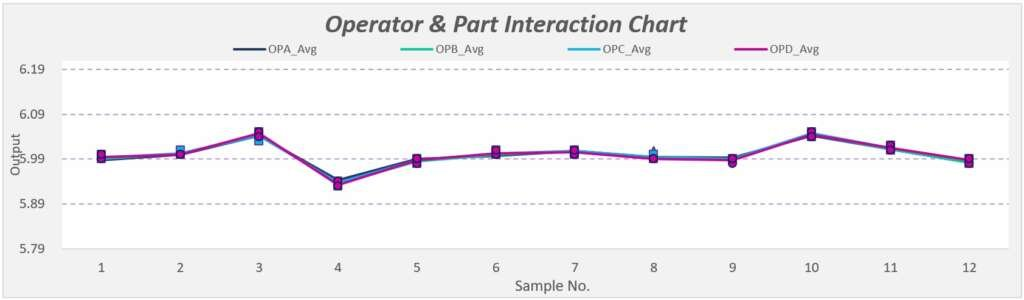Appraiser and Sample Interaction Measurement